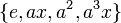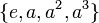# Generating sets for subgroups of dihedral group:D8

## Contents

View generating set of particular groups | View other specific information about dihedral group:D8

This article provides basic information on various choices of generating sets for subgroups of dihedral group:D8. It builds on basic information available at element structure of dihedral group:D8 and subgroup structure of dihedral group:D8.

## Probability of generation

The rule is as follows. Given$k$ (not necessarily distinct) elements picked uniformly at random and independently of each other from a finite group$G$, the probability that they all live in a fixed subgroup of index$d$ is$1/d^k$.

Using this and a form of Mobius inversion on the subgroup lattice, it is possible to compute the probability that they generate a fixed subgroup of index$d$ (we basically need to subtract off probabilities for smaller subgroups).

### Generated by one element

Here, a single element is picked uniformly at random from the group.

Automorphism class of subgroups List of all subgroups Isomorphism class Order of subgroups Index of subgroups Probability that an element is in a fixed subgroup of this automorphism class(= reciprocal of index) Probability that an element generates a fixed subgroup of this automorphism class (obtained by Mobius inversion on preceding column) Size of automorphism class Probability that the element generates a subgroup in this automorphism class
trivial subgroup$\{ e \}$ trivial group 1 8 1/8 1/8 1 1/8
center$\{ e,a^2 \}$ cyclic group:Z2 2 4 1/4 1/8 1 1/8
other subgroups of order two$\{e,x \}, \{ e,a^2x \}$$\{ e,ax \}, \{ e,a^3x \}$
cyclic group:Z2 2 4 1/4 1/8 4 1/2
Klein four-subgroups$\{ e,x,a^2,a^2x \}$,$\{ e,ax,a^2,a^3x \}$ Klein four-group 4 2 1/2 0 2 0
cyclic maximal subgroup$\{ e,a,a^2,a^3 \}$ cyclic group:Z4 4 2 1/2 1/4 1 1/4
whole group$\{ e,a,a^2,a^3,x,ax,a^2x,a^3x \}$ dihedral group:D8 8 1 1 0 1 0
Total -- -- -- -- -- -- -- 1

### Generated by two independent possibly equal elements

Here, two elements are picked uniformly at random from the group, independent of each other. They could be equal.

Automorphism class of subgroups List of all subgroups Isomorphism class Order of subgroups Index of subgroups Probability that the elements are in a fixed subgroup of this automorphism class(= reciprocal square of index) Probability that the elements generates a fixed subgroup of this automorphism class (obtained by Mobius inversion on preceding column) Size of automorphism class Probability that the elements generates a subgroup in this automorphism class
trivial subgroup$\{ e \}$ trivial group 1 8 1/64 1/64 1 1/64
center$\{ e,a^2 \}$ cyclic group:Z2 2 4 1/16 3/64 1 3/64
other subgroups of order two$\{e,x \}, \{ e,a^2x \}$$\{ e,ax \}, \{ e,a^3x \}$
cyclic group:Z2 2 4 1/16 3/64 4 3/16
Klein four-subgroups$\{ e,x,a^2,a^2x \}$,$\{ e,ax,a^2,a^3x \}$ Klein four-group 4 2 1/4 3/32 2 3/16
cyclic maximal subgroup$\{ e,a,a^2,a^3 \}$ cyclic group:Z4 4 2 1/4 3/16 1 3/16
whole group$\{ e,a,a^2,a^3,x,ax,a^2x,a^3x \}$ dihedral group:D8 8 1 1 3/8 1 3/8
Total -- -- -- -- -- -- -- 1